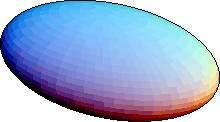Mathematics/Statistics 511 Spring 2000,
Probability.
Mathematics/Statistics 511 Spring 2000,
Probability.

# Ghosts of Classes Past:Sabbatical,
Fall 1993 and Spring 1994. *
Mathematics 141Fall 1994,
Calculus I.
* Mathematics 552Fall 1994,
Applied Complex Variables.
Mathematics 142 Spring 1995,
Calculus II.
Mathematics 141 Fall 1995,
Calculus I.
Mathematics 242 Spring 1995,
Elementary Differential Equations.
Mathematics 700 Fall 1995,
Graduate Linear Algebra.
Mathematics 142 Spring 1996,
Calculus II.
Mathematics 141 Fall 1996,
Calculus I.
* Mathematics 531 Fall 1996,
Foundations of Geometry.
Mathematics 527 Spring 1997,
Numerical Analysis.
Mathematics 122 Fall 1997,
Calculus for Business Administration
and Social Sciences.
Mathematics 723 Spring 1997,
Advanced Differential Equations.
Mathematics 524 Fall 1997,
Nonlinear Optimization.
Mathematics 550 Spring 1998,
Vector Analysis.
Mathematics 122 Fall 1998,
Calculus for Business Administration
and Social Sciences.
Mathematics 726 Spring 1998,
Graduate Numerical Analysis I.
Mathematics 727 Fall 1998,
Graduate Numerical Analysis II.
Mathematics 122 Spring 1999,
Calculus for Business Administration
and Social Sciences.
Mathematics 174 Fall 1999,
Discrete Mathematics for
Computer Science.
Mathematics 552 Spring 1999,
Applied Complex Variables.
Mathematics 700 Fall 1999,
Graduate Linear Algebra.
Mathematics 552 Spring 2000,
Applied Complex Variables.
Mathematics 748 Fall 2000,
Groups and Graphs.
Mathematics/Statistics 511 Spring 2000,
Probability.
Mathematics 174 Fall 2000,
Discrete Mathematics for
Computer Science.
Mathematics 141 Spring 2001,
Calculus I.
Mathematics 122 Fall 2001,
Calculus for Business Administration
and Social Sciences.
* Mathematics 524 Fall 2001,
Nonlinear Optimization.
Mathematics 174 Spring 2002,
Discrete Mathematics for
Computer Science.
Mathematics 122 Fall 2002,
Calculus for Business Administration
and Social Sciences.
Mathematics 527 Spring 2002,
Numerical Analysis.
Mathematics 700 Fall 2002,
Graduate Linear Algebra.
Mathematics 790 Spring 2002,
Graduate Seminar.
Mathematics 141 Spring 2003,
Calculus I.
Mathematics 141 Fall 2003,
Calculus I.
Mathematics 748 Spring 2003,
The Symmetric Group and
its Representations
Mathematics 551 Spring 2004,
Differential Geometry
Mathematics 122 Fall 2004,
Calculus for Business Administration
and Social Sciences.
Mathematics 546 Summer 2004,
Algebraic Structures I
Mathematics 705 Fall 2004,
Graduate Analysis III

Home